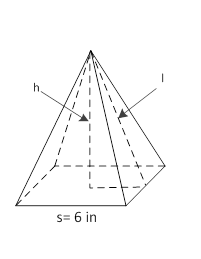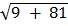### Sample Problem

The base of the following pyramid is a square. If the volume of the pyramid is 108 in3, what is its surface area? Round the answer to the nearest hundredth.The surface area = in2.

#### Solution

V = 1/3 × s × s × h

h = (3 × V) / s2  = (3 × 108) / 36 = 9 in

l == 9.49 in

So, SA = s × s + 2 × s × l

= 36 + 2 × 6 × 9.49

= 149.88 in2